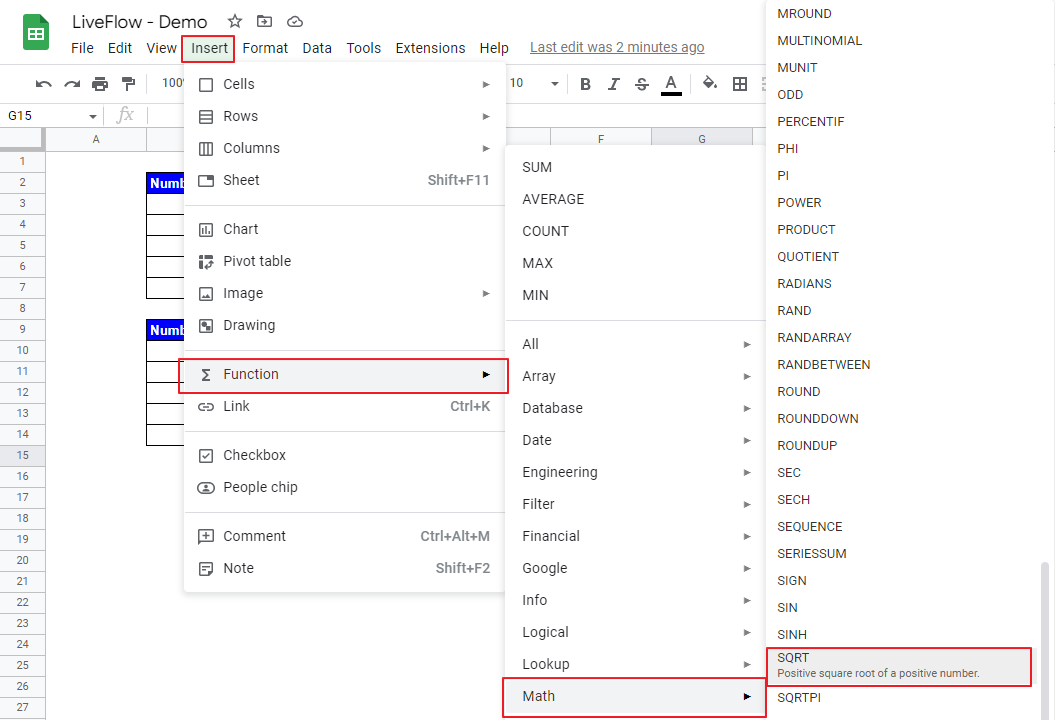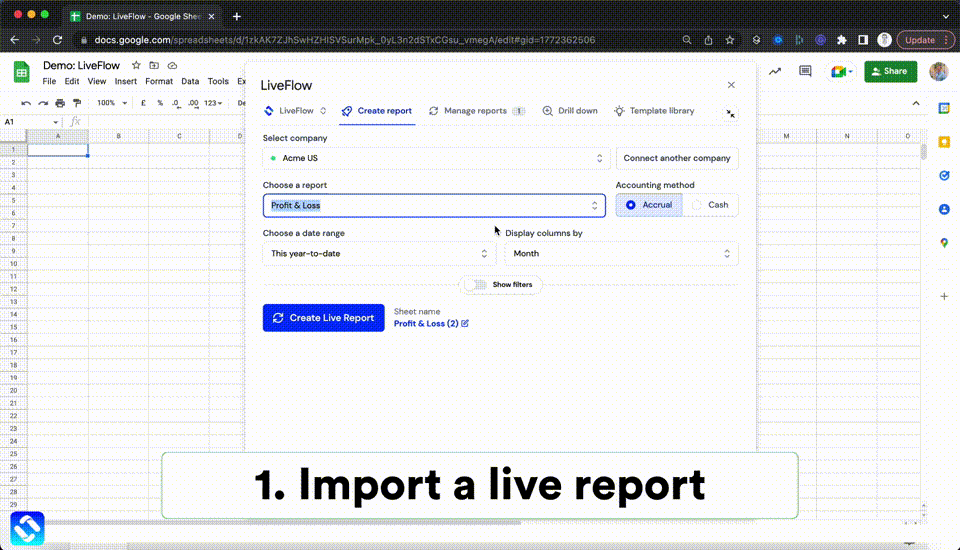# How to Find a Square Root with SQRT Function in Google Sheets

In this article, you will learn how to use the SQRT formula in Google Sheets. This formula helps you find a square root of a number easily and quickly.

## How to use the SQRT function in Google Sheets

1. Type “=SQRT(” or go to “Insert” →”Function” → ”Math” →”SQRT”.
2. Input a number, a cell, a range of cells, or a formula, for the total of which you want to find a square root.How to insert the SQRT formula from the menu bar

The generic formula is as follows:

``=SQRT(value)``

Let’s see some examples in the screenshot below. The Examples (i) show what SQRT formulas look like if they contain a number manually input. In Examples (ii), you can see formulas including single-cell references. You can understand how to incorporate a formula(s) and/or a combination of cell references in the SQRT function in Example (iii). We recommend you use cell references shown in Examples (ii) and (iii) because

## How do you do math formulas in Google Sheets?

Check this article to learn how to do basic math calculations in Google Sheets.

## How do you put a negative square root in Google Sheets?

You can get a negative square root by multiplying -1 by a result of the SQRT function. For example, if you want to find a negative square root of 25, the formula could be “=-1*SQRT(25)”.

## How do I square a number in Google Sheets?

Check this article to learn how to use the POWER formula in Google Sheets, where you see not only how to square a number but also how to compute X to the power of N by using a formula.

## Analyze your live financial data in a snap

Are you learning this formula to process financial data, build a financial model, or conduct financial analysis? If so, LiveFlow may help you to automate manual workflows and keep numbers updated in real time. For instance, you can easily compare your Budget versus Actual without any manual work. Furthermore, you can access more than fifty templates here, from the simple Income Statement and Operational KPI templates to Multi-Currency Consolidated Income Statement, Balance Sheet, and Cash Flow templates. You can also customize these templates as you want without breaking the automated data inflow.Automate any custom financial dashboard in Google Sheets with LiveFlow

Learn how to do this step-by-step in the video below 👇

## Do you need personal help?

Our team of real people are here to help you any time between 9am and 10pm GMT.Email us at: founders@liveflow.ioCall us at +1 (415) 650-1711